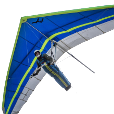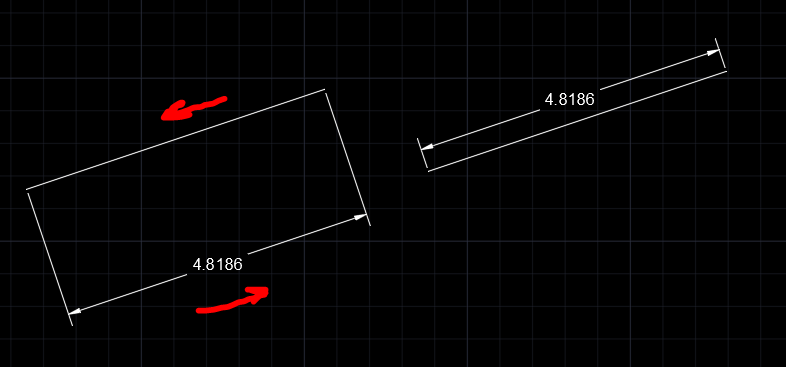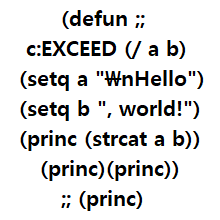# how to change distance from dimension line to objects## Recommended PostsHelp me create a lisp to change the distance from the dimension line to the object.

or is there a way to set the default distance from the dimension line to the object for each dimstyle ?

Edited by TINDANG
##### Share on other sitesMight be easier to just delete them And use/edit another lisp that creates them automatically to the right offset.

##### Share on other sites1 hour ago, mhupp said:

Might be easier to just delete them And use/edit another lisp that creates them automatically to the right offset.

Aww, I was looking for some good magic from you here! It would be a useful LISP to keep drawing output consistent.

I had a very quick look and it appears that the text position is controlled by DXF codes 10 and 11, you might be able to work something out with them and some maths maybe, though I haven't got chance today to see what you can do

•1
##### Share on other sitesTry this.

Certainly not completely as it should be, but it's a start.

So ... DXF 13 and 14 are the points to be measured.

I measure the midppoint between those points, then DXF 10 and 11 are set 30 units (the user sets this) from that midpoint, perpendicular to the line 13 - 14.

So for the moment it's for aligned dimentions, it won't work in all conditions.

Command DFD

```
(/ (* PI ang) 180.0)
)

(defun rad2deg ( ang / )
(/ (* 180.0 ang) PI)
)

;; midpoint of 2 given points
(defun mid ( pt1 pt2 / )
(mapcar '(lambda (x y) (+ (* 0.5 x) (* 0.5 y)))
pt1
pt2
)
)

(defun c:dfd ( / dist sel ent al ar ang1 ang2 mp tp txp)

(princ "\nDistance DIM: ")
(setq dist (getreal))

(while (setq sel (entsel "\nSelect DIM: "))
(setq ent (car sel ))

(setq txp (cdr (assoc 10 (entget ent))))
(setq al (cdr (assoc 13 (entget ent))))
(setq ar (cdr (assoc 14 (entget ent))))
(setq ang1 (angle al ar))
(setq mp (mid al ar))

(setq ang2 (angle mp txp))

(if (< ang1 ang2)
(setq tp (polar mp (+ ang1 (deg2rad 90.0)) dist))
(setq tp (polar mp (- ang1 (deg2rad 90.0)) dist))
)
(entmod (subst (cons 10 tp) (assoc 10 (entget ent)) (entget ent) ))
(entmod (subst (cons 11 tp) (assoc 11 (entget ent)) (entget ent) ))
)
(princ)

)```

Edited by Emmanuel Delay
•1
##### Share on other sitesTo have angle 90 Deg. in Radians. e.g:

```(* pi 0.5)
;; or
(/ pi 2.0) ```

##### Share on other sites@Emmanuel Delay your code places the dimension on the opposite side of the line when ar is to the left of al and the aligned dimension goes from left to right.

Here's a before and after shot.I avoid using angles although sometimes it is easier.  Here's a modified version of your code using vectors.

```(defun deg2rad (ang / )
(/ (* PI ang) 180.0)
)

(defun rad2deg ( ang / )
(/ (* 180.0 ang) PI)
)

;; midpoint of 2 given points
(defun mid ( pt1 pt2 / )
(mapcar '(lambda (x y) (+ (* 0.5 x) (* 0.5 y)))
pt1
pt2
)
)
;;;  Calculate unit vector of vector a
(defun uvec
(a / d)
(setq	d (distance '(0 0 0) a)
a (mapcar '/ a (list d d d))
)
)
; Compute the dot product of 2 vectors a and b
(defun dot ( a b / dd)
(setq dd (mapcar '* a b))
(setq dd (+ (nth 0 dd) (nth 1 dd) (nth 2 dd)))
)					;end of dot

(defun c:test2 ( / sel ent txp al ar mp uALR s txpp uvt tp )

(princ "\nDistance DIM: ")
(setq dist (getreal))

(while (setq sel (entsel "\nSelect DIM: "))
(setq ent (car sel ))

(setq txp (cdr (assoc 10 (entget ent))))
(setq al (cdr (assoc 13 (entget ent))))
(setq ar (cdr (assoc 14 (entget ent))))
;;;	  (setq ang1 (angle al ar))
;;;	  (setq mp (mid al ar))
;;;
;;;	  (setq ang2 (angle mp txp))
;;;
;;;	  (if (< ang1 ang2)
;;;		(setq tp (polar mp (+ ang1 (deg2rad 90.0)) dist))
;;;		(setq tp (polar mp (- ang1 (deg2rad 90.0)) dist))
;;;	  )
(setq mp (mapcar '/
(mapcar '+ al ar)
'(2. 2. 2.)
)
)
; uALR = unit vector from al to ar
(setq uALR (uvec (mapcar '- ar al)))
(setq s (dot uALR (mapcar '- txp al)))
; txpp = projection of txp onto the line
(setq txpp
(mapcar '+ al (mapcar '* uALR (list s s s)))
)
(setq uvt (uvec (mapcar '- txp txpp)))
(setq tp (mapcar '+ mp (mapcar '* uvt (list dist dist dist))))
(entmod
(subst (cons 10 tp) (assoc 10 (entget ent)) (entget ent))
)
(entmod
(subst (cons 11 tp) (assoc 11 (entget ent)) (entget ent))
)
)
(princ)
)```

•4
##### Share on other sitesPlease give it a test , maybe some defun is miss, it only work for "AcDbAlignedDimension"

Feel free to add error handling or what else ......

```;************************************************************

;; Design by Gabo CALOS DE VIT from CORDOBA ARGENTINA
;;;    Copyleft 1995-2022 by Gabriel Calos De Vit ; DEVITG@GMAIL.COM
;;
; ----------------------------------------------------------------------
; DISCLAIMER:  Gabriel Calos De Vit Disclaims any and all liability for any damages
; arising out of the use or operation, or inability to use the software.
; FURTHERMORE, User agrees to hold Gabriel Calos De Vit harmless from such claims.
; Gabriel Calos De Vit makes no warranty, either expressed or implied, as to the
; fitness of this product for a particular purpose.  All materials are
; to be considered ‘as-is’, and use of this software should be
; considered as AT YOUR OWN RISK.
; ----------------------------------------------------------------------

;;************************************************************

;*//*/*/*/*/*/*/*/*/*/*/*/*/**/*/*/*/*/*/*/*/*/*/*/*/*/*/*/*/*/*/*/*/*/*/*/*/*/*/*/*/*/*/*/*
(DEFUN &-TEXT/STR-PT-HEI  (STR PT HEIG) ;_ 01
(IF (= (TYPE PT) 'LIST)
(SETQ P1 (VLAX-3D-POINT PT))
(SETQ P1 PT)
)
)
;;;;-*******************************************************************************************************************************
;;*//*/*/*/*/*/*/*/*/*/*/*/*/**/*/*/*/*/*/*/*/*/*/*/*/*/*/*/*/*/*/*/*/*/*/*/*/*/*/*/*/*/*/*/*
(DEFUN G-MIDPOINT/P1-P2  (P1 P2) ;_01
(MAPCAR '* '(0.5 0.5 0.5) (MAPCAR '+ P1 P2))
)
;;*//*/*/*/*/*/*/*/*/*/*/*/*/**/*/*/*/*/*/*/*/*/*/*/*/*/*/*/*/*/*/*/*/*/*/*/*/*/*/*/*/*/*/*/*

;;*//*/*/*/*/*/*/*/*/*/*/*/*/**/*/*/*/*/*/*/*/*/*/*/*/*/*/*/*/*/*/*/*/*/*/*/*/*/*/*/*/*/*/*/*
(DEFUN VAR->LST  (VARIANT#) ;_01
(VLAX-SAFEARRAY->LIST (VLAX-VARIANT-VALUE VARIANT#))
)
;;*//*/*/*/*/*/*/*/*/*/*/*/*/**/*/*/*/*/*/*/*/*/*/*/*/*/*/*/*/*/*/*/*/*/*/*/*/*/*/*/*/*/*/*/*

;;;arrange-dim-text-position
;;;*************************************************************;;;

(defun arrange-dim-text-position (/

EXTLINE1POINT-VAR EXTLINE1POINT-XYZ EXTLINE2POINT-VAR
EXTLINE2POINT-XYZ MID-PT1-PT2 MODEL NEW-TEXTPOSITION-XYZ NO-WAY
TEXTPOSITION-ANGLE TEXTPOSITION-OBJ TEXTPOSITION-VAR
TEXTPOSITION-XYZ
) ;_  /

(initget 7)
(if (not
(setq desired-dist (getreal "distance from side"))
) ;_  not
(setq desired-dist 15.0)
) ;_  if
(setq dim-ent-ss (ssget "_X" '((0 . "dim*"))))

;;;  (setq DIM-OBJ (vla-Item dim-obj-ss 0))
(vlax-for
dim-obj dim-obj-ss
(if(= (vla-get-ObjectName dim-obj) "AcDbAlignedDimension")

(progn
(setq ExtLine1Point-var (VLA-GET-ExtLine1Point dim-obj))
(setq ExtLine1Point-xyz (VAR->LST ExtLine1Point-var))
(setq ExtLine2Point-var (VLA-GET-ExtLine2Point dim-obj))
(setq ExtLine2Point-xyz (VAR->LST ExtLine2Point-var))
(setq mid-pt1-pt2 (G-MIDPOINT/P1-P2 ExtLine2Point-xyz ExtLine1Point-xyz))
(setq TextPosition-var (vla-get-TextPosition dim-obj))
(setq TextPosition-xyz (VAR->LST TextPosition-var))
(setq TextPosition-angle (angle mid-pt1-pt2 TextPosition-xyz))
(setq new-TextPosition-xyz (polar mid-pt1-pt2 TextPosition-angle desired-dist))
(vla-put-TextPosition dim-obj (VLAX-3D-POINT new-TextPosition-xyz))
);end progn
(progn ;for no way to move

(setq TextPosition-obj (vla-get-TextPosition dim-obj))
(setq no-way ( &-TEXT/STR-PT-HEI "NO-way " TextPosition-obj (* 2 (vla-get-TextHeight dim-obj))))
(vla-put-color no-way acred  )
);end prog for no way to move

);end if
) ;_  vlax-for

) ;end defun

(defun c:arr-dim ()

(arrange-dim-text-position)

)

;;;(200 2 1 0 nil "end of " 100 20 2 2 nil nil T nil T)
;;;;*** DO NOT add text below the comment! ***|;
```

•1
##### Share on other sites@lrm, thanks, your code worked great. But in case of sizes with different length extension lines doesn't work. Can you help me?

Edited by TINDANG
##### Share on other sitesIs it quicker select object ask for dim offset and just do all dims brand new so no mistakes ?

##### Share on other sites9 hours ago, TINDANG said:

@lrm, thanks, your code worked great. But in case of sizes with different length extension lines doesn't work. Can you help me?

I did the lisp as the  the image post.

I have as My personal rule , to work on the ORIGINAL image or sample.dwg , and never make any GUESS, neither ask to an ORACLE what the OP what he could done or will do .

##### Share on other sites14 hours ago, TINDANG said:

@lrm, thanks, your code worked great. But in case of sizes with different length extension lines doesn't work. Can you help me?

I did the lisp as the  the image post.

I have as My personal rule , to work on the ORIGINAL image or sample.dwg , and never make any GUESS, neither ask to an ORACLE what the OP what he could done or will do .

##### Share on other sitesQuote

I did the lisp as the  the image post.

I have as My personal rule , to work on the ORIGINAL image or sample.dwg , and never make any GUESS, neither ask to an ORACLE what the OP what he could done or will do .

It looks like you did guess about the direction of the line segment and the sequence of the dimensioned vertices.  E.g.,  reverse the direction  of the polyline in your test drawing "arrange dim tests.dwg".  It is best to to minimize guesses about an OP's post but I have found many are in need of some interpretation.

##### Share on other sites@devitg  It looks like we both assumed that the dimensions were aligned.  Do you have a clean way to determine if a dimension line is linear vs. aligned?

##### Share on other sites@Irm , at this case I broke my own rule , a made a dwg from trash.

About how to know what dimension kind  is I use the

`(VLAX-DUMP-OBJECT dim-obj t)`

Because  Enget give 4 different  DXF 100 code for the same dim .

Quote

("AcDbEntity" "AcDbDimension" "AcDbAlignedDimension" "AcDbRotatedDimension")

but the DUMP

Quote

(VLAX-DUMP-OBJECT dim-obj t)

give only one

Quote

ObjectName (RO) = "AcDbRotatedDimension"

```(vla-get-ObjectName dim-obj)

```

##### Share on other sites

• 3 weeks later...On 1/22/2022 at 2:45 AM, lrm said:

@Emmanuel Delay your code places the dimension on the opposite side of the line when ar is to the left of al and the aligned dimension goes from left to right.

Here's a before and after shot.

I avoid using angles although sometimes it is easier.  Here's a modified version of your code using vectors.

```(defun deg2rad (ang / )
(/ (* PI ang) 180.0)
)

(defun rad2deg ( ang / )
(/ (* 180.0 ang) PI)
)

;; midpoint of 2 given points
(defun mid ( pt1 pt2 / )
(mapcar '(lambda (x y) (+ (* 0.5 x) (* 0.5 y)))
pt1
pt2
)
)
;;;  Calculate unit vector of vector a
(defun uvec
(a / d)
(setq	d (distance '(0 0 0) a)
a (mapcar '/ a (list d d d))
)
)
; Compute the dot product of 2 vectors a and b
(defun dot ( a b / dd)
(setq dd (mapcar '* a b))
(setq dd (+ (nth 0 dd) (nth 1 dd) (nth 2 dd)))
)					;end of dot

(defun c:test2 ( / sel ent txp al ar mp uALR s txpp uvt tp )

(princ "\nDistance DIM: ")
(setq dist (getreal))

(while (setq sel (entsel "\nSelect DIM: "))
(setq ent (car sel ))

(setq txp (cdr (assoc 10 (entget ent))))
(setq al (cdr (assoc 13 (entget ent))))
(setq ar (cdr (assoc 14 (entget ent))))
;;;	  (setq ang1 (angle al ar))
;;;	  (setq mp (mid al ar))
;;;
;;;	  (setq ang2 (angle mp txp))
;;;
;;;	  (if (< ang1 ang2)
;;;		(setq tp (polar mp (+ ang1 (deg2rad 90.0)) dist))
;;;		(setq tp (polar mp (- ang1 (deg2rad 90.0)) dist))
;;;	  )
(setq mp (mapcar '/
(mapcar '+ al ar)
'(2. 2. 2.)
)
)
; uALR = unit vector from al to ar
(setq uALR (uvec (mapcar '- ar al)))
(setq s (dot uALR (mapcar '- txp al)))
; txpp = projection of txp onto the line
(setq txpp
(mapcar '+ al (mapcar '* uALR (list s s s)))
)
(setq uvt (uvec (mapcar '- txp txpp)))
(setq tp (mapcar '+ mp (mapcar '* uvt (list dist dist dist))))
(entmod
(subst (cons 10 tp) (assoc 10 (entget ent)) (entget ent))
)
(entmod
(subst (cons 11 tp) (assoc 11 (entget ent)) (entget ent))
)
)
(princ)
)```

I often use this routine, after Dimensioning Multiple Segments of a Polyline Lisp (DPI DPO).

so, this is just little bit minor change of rlm's code, edit entsel to ssget

for do this routine to multiple objects in one procedure

```(defun c:test2 ( / sel ent txp al ar mp uALR s txpp uvt tp exss exssent exssl exssindex)
(/ (* PI ang) 180.0)
)

(defun rad2deg ( ang / )
(/ (* 180.0 ang) PI)
)

;; midpoint of 2 given points
(defun mid ( pt1 pt2 / )
(mapcar '(lambda (x y) (+ (* 0.5 x) (* 0.5 y)))
pt1
pt2
)
)
;;;  Calculate unit vector of vector a
(defun uvec
(a / d)
(setq	d (distance '(0 0 0) a)
a (mapcar '/ a (list d d d))
)
)
; Compute the dot product of 2 vectors a and b
(defun dot ( a b / dd)
(setq dd (mapcar '* a b))
(setq dd (+ (nth 0 dd) (nth 1 dd) (nth 2 dd)))
)					;end of dot

(princ "\nDistance DIM: ")
(setq dist (getreal))

(princ "\nSelect DIM: ")                                    ;edited line
(setq exss (ssget '((0 . "*dim*"))))                           ;edited line
(setq exssl (sslength exss))                                   ;edited line
(setq exssindex 0)                                             ;edited line
(repeat exssl                                                    ;edited line
(setq exssent (entget (ssname exss exssindex)))         ;edited line

;(while (setq sel (entsel "\nSelect DIM: "))                ;edited line
;	  (setq ent (car sel ))                               ;edited line

(setq ent (cdr (car exssent)))                                 ;edited line
(setq txp (cdr (assoc 10 (entget ent))))
(setq al (cdr (assoc 13 (entget ent))))
(setq ar (cdr (assoc 14 (entget ent))))
;;;	  (setq ang1 (angle al ar))
;;;	  (setq mp (mid al ar))
;;;
;;;	  (setq ang2 (angle mp txp))
;;;
;;;	  (if (< ang1 ang2)
;;;		(setq tp (polar mp (+ ang1 (deg2rad 90.0)) dist))
;;;		(setq tp (polar mp (- ang1 (deg2rad 90.0)) dist))
;;;	  )
(setq mp (mapcar '/
(mapcar '+ al ar)
'(2. 2. 2.)
)
)

; uALR = unit vector from al to ar
(setq uALR (uvec (mapcar '- ar al)))
(setq s (dot uALR (mapcar '- txp al)))
; txpp = projection of txp onto the line
(setq txpp
(mapcar '+ al (mapcar '* uALR (list s s s)))
)
(setq uvt (uvec (mapcar '- txp txpp)))
(setq tp (mapcar '+ mp (mapcar '* uvt (list dist dist dist))))
(entmod
(subst (cons 10 tp) (assoc 10 (entget ent)) (entget ent))
)
(entmod
(subst (cons 11 tp) (assoc 11 (entget ent)) (entget ent))
)
;)                                           ;edited line while delted
(setq exssindex (+ exssindex 1))    ;edited line for repeat added
)                                           ;edited line for repeat added
(princ)
)
```

•1

## Join the conversation

You can post now and register later. If you have an account, sign in now to post with your account.
Note: Your post will require moderator approval before it will be visible.×   Pasted as rich text.   Restore formatting

Only 75 emoji are allowed.